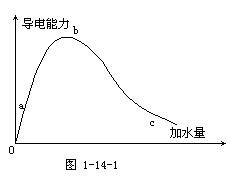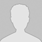#学习小专题

## 从九个方面关注离子浓度大小比较问题

1 关注三个守恒

c(H3O+)+c(H2CO3)=c(NH3)+c(OH-)+c(CO32-)

1Na2S溶液中下列关系不正确的是

A． c(Na+) =2c(HS) +2c(S2) +c(H2S)

Bc(Na+) +c(H+)=c(OH)+c(HS)+2c(S2)

Cc(Na+)c(S2)c(OH)c(HS

Dc(OH)=c(HS)+c(H+)+c(H2S)

2 关注混合后溶液的酸碱性

2 下列混合溶液中，各离子浓度的大小顺序正确的是

A 10mL0.1mol・L1氨水与10mL0.1mol・L1盐酸混合：

c(Cl)>c(NH4+)>c(OH)>c(H+)

B10mL0.1mol・L1NH4Cl5mL0.2mol・L1NaOH溶液混合：c(Na+)=c(Cl-)>c(OH-)>c(H+)

C10mL0.1mol・L1CH3COOH溶液与5mL0.2mol・L1NaOH溶液混合：

c(Na+)=c(CH3COO-)>c(OH-)>c(H+)

D10mL0.5mol・L1CH3COONa溶液与6mL1mol・L1盐酸混合：

c(Cl-)>c(Na+)>c(OH-)>c(H+)

30.2mol・L1HCN溶液和0.1mol・L1NaOH溶液等体积混合后，溶液显碱性，下列关系式中正确的是

A. c(HCN)<c(CN)                B. c(Na)>c(CN)

C. c(HCN)c(CN)c(OH)       D. c(HCN)c(CN)0.1mol・L1

3 关注用来表示溶液中的溶质

4 物质的量浓度相同的200mL Ba(OH)2溶液和150mL NaHCO3混合后，最终溶液中离子的物质的量浓度关系正确的是

A.     c(OH)>c(Ba2+)>c(Na+)>c(CO32) B. c(OH)>c(Na+)>c(Ba2+)>C(H+)

C. c(OH)=c(Ba2+)+c(Na+)+c(H+)       D. c(H+)+c(Na+)+2c(Ba2+)=c(OH)

4 关注所给物质的量是物质的量浓度还是pH

在审题时，要关注所给物质的量是物质的量浓度还是“pH”，否则会很容易判断错误。

5设氨水的pHx，某盐酸的pHy，已知x+y14，且x>11。将上述两溶液分别取等体积充分混合后，所得溶液中各离子浓度由大到小的顺序是

A. c(Cl)>c(NH4+)>c(H+)>c(OH)    B. c(NH4+)>c(Cl)>c(H+)>c(OH)

C. c(NH4+)>c(Cl)>c(OH)>c(H+)    D. c(Cl)>c(NH4+)>c(OH)>c(H+)

5 关注所给酸与碱的相对强弱

对于未指明所给的酸和碱的相对强弱，要考虑两种情况，一是强电解质，二是弱电解质。

6一元酸HA溶液中，加入一定量强碱MOH溶液后，恰好完全反应，反应后的溶液中，下列判断正确的是(     )

A[A-]≥[M+]                                  B[A-]≤[M+]

C．若MA不水解,[OH-]<[A-]     D．若MA水解,[OH-]>[A-]

6关注弱酸弱碱的稀释问题7用水稀释0.1mol・L1氨水时，溶液中随着水量的增加而减小的是

A. c(OH)/c(NH3・H2O)                          B. c(NH3・H2O)/c(OH

C. c(H+)c(OH)的乘积                       D. OH的物质的量

7 关注利用溶液中阴阳离子的种类来判断溶液中溶质的可能情况

8已知某无色透明的溶液中所含的离子仅有Na+CH3COO-H+OH-四种离子，试回答下列问题：

A. c(Na+)>c(CH3COO)>c(OH)>c(H+)    B. c(CH3COO)=c(Na+)>c(OH)=c(H+)

C. c(H+)>c(OH)>c(Na+)>c(CH3COO)     D. c(Na+)>c(CH3COO)>c(H+)>c(OH)

8关注未知二元酸的电离情况

对所给的二元酸的电离要关注电离的程度：是否完全电离。

9某二元酸（化学式用H2A表示）在水中的电离方程式是：

H2A==H++HAHAH++A2 回答下列问题：

Na2A溶液显   （填酸性中性，或碱性）。

0.1mol・L1Na2A溶液中，下列微粒浓度关系式正确的是：

A. c(A2) +c( HA)+c( H2A) =0.1mol・L-1         B. c(OH)=c(H+) +c( HA)

C. c(Na+ )+ c(H+) =c(OH)+ c( HA)+2c(A2)     D. c(Na+ )= 2c(A2) +2c( HA)

⑶已知0.1mol・L1NaHA溶液的pH=2，则0.1mol・L-1 H2A溶液中氢离子的物质的量浓度可能是          0.11 mol・L1（填“<”，“>”，或“=”），理由是：

⑷0.1mol・L1 NaHA溶液中各种离子浓度由大到小的顺序是：

9关注隐含信息的发掘

10今有一混合物的水溶液，只可能含有以下离子中的若干种：K+NH4+ClMg2+Fe3+CO32―SO42―，现每次取100.00ml进行实验。

⑴判断K+、Cl是否存在？K+                       ；Cl                     （填下列序号）。

A．一定存在          B．可能存在          C．一定不存在

⑵肯定存在的离子的原溶液中的物质的量浓度分别为

(责任编辑：)

------分隔线----------------------------

------分隔线----------------------------

 离子浓度大小比较之三大守恒专题 离子浓度大小比较之分布曲线专练 离子浓度大小比较之水的电离曲线 离子浓度大小比较之三大守恒专题 离子浓度大小比较之强碱滴定二元 离子浓度大小比较之对数曲线专练 用好“四点”突破图像类溶液中微 微课视频之乐乐课堂：盐类水解反 常见盐溶液中离子浓度的精准算法 2008～2017江苏高考离子浓度比较 离子浓度的大小比较专题文档 微课视频：溶液中粒子浓度大小比 微课视频：离子浓度大小比较 电解质溶液中微粒浓度大小比较的 溶液中离子浓度大小比较的常见错 溶液中离子浓度大小的比较及其规 电解质溶液稀释时离子浓度的变化 离子浓度大小比较一、二、三、四• 全部评论（0
还没有评论，快来抢沙发吧！